# The yield to maturity and bond equivalent yield

Get familiar with how rates of return are calculated, including one of the most common measures—the yield to maturity.

•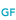WILEY GLOBAL FINANCE

The financial markets serve as conduits through which funds are distributed from borrowers to lenders. The allocation of funds is determined by the relative rates paid on bonds, loans, and other financial securities, with the differences in rates among claims being determined by risk, maturity, and other factors that serve to differentiate the claims. There are a number of different measures of the rates of return on bonds and loans. Some measures, for example, determine annual rates based on cash flows received over 365 days, whereas others use 360 days; some measures determine rates that include the compounding of cash flows, whereas some do not; and some measures include capital gains and losses, whereas others exclude price changes. In this section, we examine some of the measures of rates of return, including the most common measure—the yield to maturity.

## Common measures of rates of return

When the term rate of return is used it can mean a number of different rates, including the interest rate, coupon rate, current yield, or discount yield. The term interest rate sometimes refers to the price a borrower pays a lender for a loan. Unlike other prices, this price of credit is expressed as the ratio of the cost or fee for borrowing and the amount borrowed. This price is typically expressed as an annual percentage of the loan (even if the loan is for less than one year). Today, financial economists often refer to the yield to maturity on a bond as the interest rate. In this article, the term interest rate will mean yield to maturity.

Another measure of rate of return is a bond's coupon rate. As noted in the last section, the coupon rate, CR, is the contractual rate the issuer agrees to pay each period. It is usually expressed as a proportion of the annual coupon payment to the bond's face value: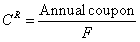Unless the bond is purchased at par, the coupon rate is not a good measure of the bond's rate of return because it fails to take into account the price paid for the bond.

In examining corporate bond quotes, the current yield on a bond is often provided. As noted, this rate is computed as the ratio of the bond's annual coupon to its current price. This measure provides a quick estimate of a bond's rate of return, but in many cases not an accurate one because it does not capture price changes. The current yield is a good approximation of the bond's yield, if the bond is selling at or near its face value or if it has a long maturity. That is, we noted earlier that if a bond is selling at par, its coupon rate is equal to the discount rate. In this case, the current yield is equal to the bond's yield to maturity. Thus, the closer the bond's price is to its face value, the closer the current yield is to the bond's yield to maturity. As for maturity, note that a coupon bond with no maturity or repayment of principal, known as a perpetuity or consul, pays a fixed amount of coupons forever. The value of such a bond is: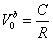If the bond is priced in the market to equal Vbo, then the rate on the bond would be equal to the current yield: R = C/ Vbo. Thus, when a coupon bond has a long-term maturity (e.g., 20 years), then it is similar to a perpetuity, making its current yield a good approximation of its rate of return.

Finally, the discount yield is the bond's return expressed as a proportion of its face value. For example, a one-year zero-coupon bond costing \$900 and paying a par value of \$1,000 yields \$100 in interest and a discount yield of 10%: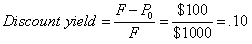The discount yield used to be the rate frequently quoted by financial institutions on their loans (because the discount rate is lower than a rate quoted on the borrowed amount). The difficulty with this rate measure is that it does not capture the conceptual notion of the rate of return being the rate at which the investment grows. In this example, the \$900 bond investment grew at a rate of over 11%, not 10%: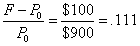Because of tradition, the rates on Treasury bills are quoted by dealers in terms of the bills' discount yields. Whereas Treasury bills have maturities less than one year, the discount yields are quoted on an annualized basis. As we noted in the last section, dealers quoting the annualized rates use a day count convention of actual days to maturity but with a 360-day year: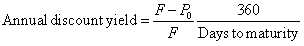## Yield to maturity

The most widely used measure of a bond's rate of return is the yield to maturity (YTM). As noted earlier, the YTM, or simply the yield, is the rate that equates the purchase price of the bond with the present value of its future cash flows. Mathematically, the YTM (y) is found by solving the following equation: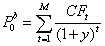Equation (1)

The YTM is analogous to the internal rate of return used in capital budgeting. It is a measure of the rate at which the investment grows. From our first example, if the 10-year, 9% annual coupon bond were actually trading in the market for \$938.55, then the YTM on the bond would be 10%. Unlike the current yield, the YTM incorporates all of the bond's cash flows (CFs). It also assumes the bond is held to maturity and that all CFs from the bond are reinvested to maturity at the calculated YTM.

## Estimating YTM: average rate to maturity

If the cash flows on the bond (coupons and principal) are not equal, then Equation (1) cannot be solved directly for the YTM. Alternatively, one must use an iterative (trial and error) procedure, substituting different y values into Equation (1) until a y is found that equates the present value of the bond's cash flows to the market price. An estimate of the YTM, however, can be found using the bond's average rate to maturity, ARTM (also referred to as the yield approximation formula). This measure determines the rate as the average return per year as a proportion of the average price of the bond per year. For a coupon bond with a principal paid at maturity, the average return per year on the bond is its annual coupon plus its average annual capital gain. For a bond with an M-year maturity, its average gain is calculated as the total capital gain realized at maturity divided by the number of years to maturity: (F - Pbo)/M. The average price of the bond is computed as the average of 2 known prices, the current price and the price at maturity (F): (F + Pbo)/2. Thus the ARTM is: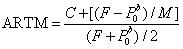The ARTM for the 10-year, 9% annual coupon bond trading at \$938.55 is 0.0992: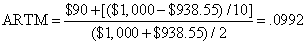## Bond equivalent yields

The YTM calculated above represents the yield for the period (in the above example this was an annual rate, given annual coupons). If a bond's CFs were semiannual, then solving Equation (1) for y would yield a 6-month rate; if the CFs were monthly, then solving (1) for y would yield a monthly rate. To obtain a simple annualized rate (with no compounding), yA, one needs to multiply the periodic rate, y, by the number of periods in the year. Thus, if a 10-year bond paying \$45 every 6 months and \$1,000 at maturity were selling for \$937.69, its 6-month yield would be .05 and its simple annualized rate, yA, would be 10%: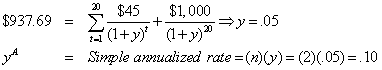In this example, the simple annualized rate is obtained by determining the periodic rate on a bond paying coupons semiannually and then multiplying by 2. Because Treasury bonds and many corporate bonds pay coupons semiannually, the rate obtained by multiplying the semiannual periodic rate by 2 is called the bond-equivalent yield. Bonds with different payment frequencies often have their rates expressed in terms of their bond-equivalent yields so that their rates can be compared to each other on a common basis. This bond-equivalent yield, though, does not take into account the reinvestment of the bond's cash flows during the year. Therefore, it underestimates the actual rate of return earned. Thus, an investor earning 5% semiannually would have \$1.05 after 6 months from a \$1 investment that they can reinvest for the next 6 months. If they reinvest at 5%, then their annual rate would be 10.25% [= (1.05)(1.05) - 1 = (1.05)2 - 1], not 10%. As noted earlier, the 10.25% annual rate, which takes into account compounding, is known as the effective rate.